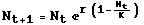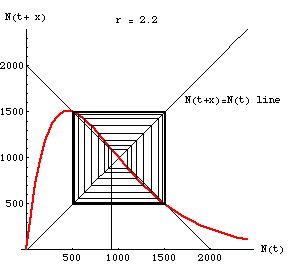ZOO 4400/5400 Population Ecology

Intraspecific competiton -- discrete logistic equation
high r 2-point cycle caseEqn 15.1

Embedded QuickTime Movie (from Mathematica notebook DiscLogAnimTraj03.nb)

Click on the image below to start the "movie". A control bar will appear below the graph; the various buttons will allow you to stop and start it.

Fig. 1. One-dimensional "map" of the discrete logistic (Eqn 15.1, above) with r = 2.2 and K = 1,000. Note that the population converges on a two-point cycle. We start with an N(t) value on the X-axis, move up to the "map" (red line) to get an N(t+x) value and then take the corresponding Y-value by going over to the 1:1 black line, then again move to the red line. Tracing the map is therefore a process of going back and forth from the red map line to the black N(t+x) = N(t) 1:1 line (like a box step dance -- "up, right, down, left"). Following the map this way gives us the successive population sizes over time. Note that the trajectory moves away from the unstable equilibrium point (at K = 1,000) and toward an oscillation between two other stable equilibrium points -- one at 497 and the other at 1,503. Once it gets there it will oscillate endlessly between these two values.

The high r value here is what drives the cycle.Fig. 2. Static view of the trajectory toward the 2-point stable equilibrium from a starting N0 of 950.
Note the rectangular outward spiral from the starting value (near the unstable equilibrium point K) to the stable oscillatory points, 497 and 1503.  The upper left corner is at {497, 1503} while the lower right is {1503, 497}. These are the two places the red map curve crosses the line of slope -1 (it also crosses at K).